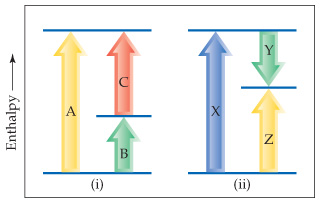# Problem: Consider the two diagrams below.Equation from Part A: ΔHA = ΔHB + ΔHCEquation from Part B: ΔHZ = ΔHX + ΔHYThe equations you obtained in parts (a) and (b) are based on what law?a) Hess's Law b) Charles' Law c) Conservation of mass d) Boyle's Law

###### FREE Expert Solution

The equations show the relationships between the individual enthalpies of each reaction as the sum of an  overall reaction equation.

92% (261 ratings)###### Problem Details

Consider the two diagrams below.• Equation from Part A: ΔHA = ΔHB + ΔHC
• Equation from Part B: ΔHZ = ΔHX + ΔHY

The equations you obtained in parts (a) and (b) are based on what law?

a) Hess's Law

b) Charles' Law

c) Conservation of mass

d) Boyle's Law